# Unit-7.pdf

21 Dec 2022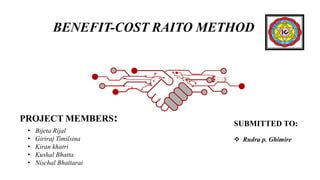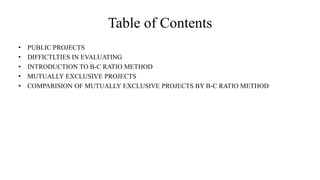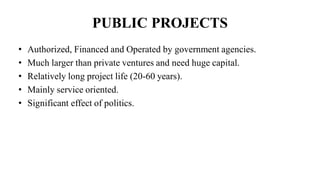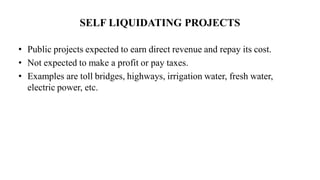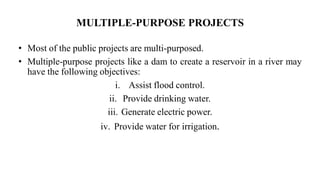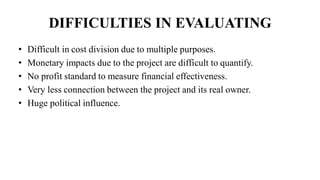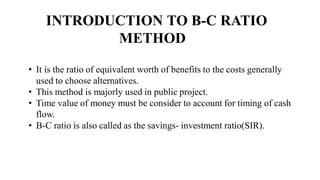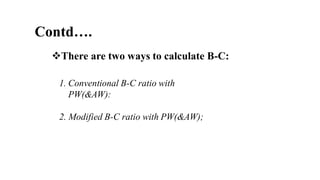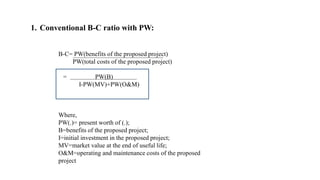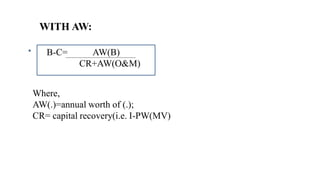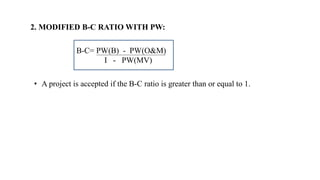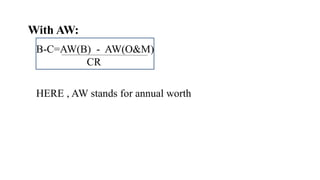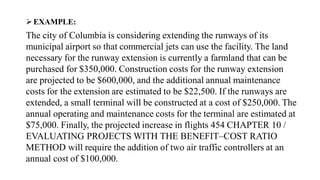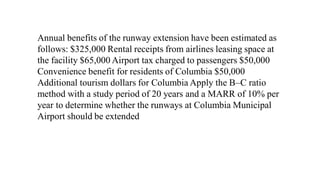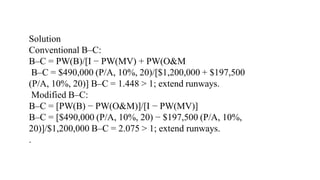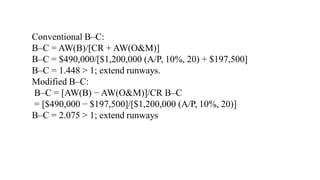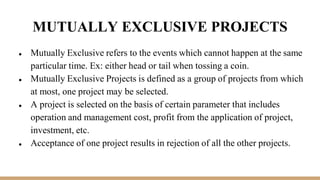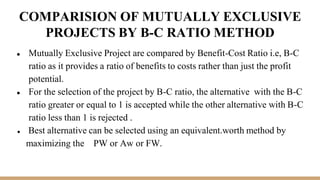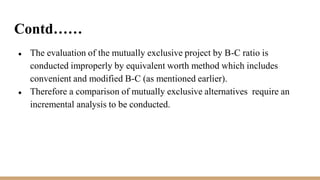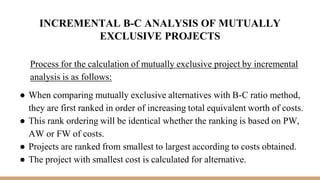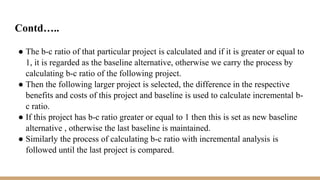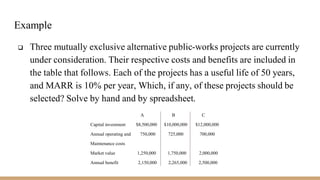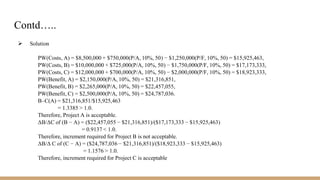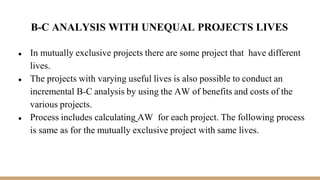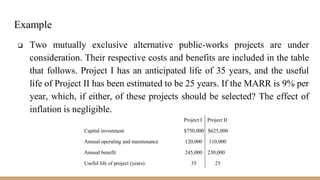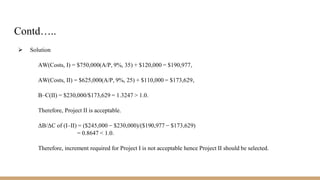1 sur 27

### Unit-7.pdf

• 1. BENEFIT-COST RAITO METHOD PROJECT MEMBERS: • Bijeta Rijal • Giriraj Timilsina • Kiran khatri • Kushal Bhatta • Nischal Bhattarai SUBMITTED TO:  Rudra p. Ghimire
• 2. Table of Contents • PUBLIC PROJECTS • DIFFICTLTIES IN EVALUATING • INTRODUCTION TO B-C RATIO METHOD • MUTUALLY EXCLUSIVE PROJECTS • COMPARISION OF MUTUALLY EXCLUSIVE PROJECTS BY B-C RATIO METHOD
• 3. PUBLIC PROJECTS • Authorized, Financed and Operated by government agencies. • Much larger than private ventures and need huge capital. • Relatively long project life (20-60 years). • Mainly service oriented. • Significant effect of politics.
• 4. SELF LIQUIDATING PROJECTS • Public projects expected to earn direct revenue and repay its cost. • Not expected to make a profit or pay taxes. • Examples are toll bridges, highways, irrigation water, fresh water, electric power, etc.
• 5. MULTIPLE-PURPOSE PROJECTS • Most of the public projects are multi-purposed. • Multiple-purpose projects like a dam to create a reservoir in a river may have the following objectives: i. Assist flood control. ii. Provide drinking water. iii. Generate electric power. iv. Provide water for irrigation.
• 6. DIFFICULTIES IN EVALUATING • Difficult in cost division due to multiple purposes. • Monetary impacts due to the project are difficult to quantify. • No profit standard to measure financial effectiveness. • Very less connection between the project and its real owner. • Huge political influence.
• 7. INTRODUCTION TO B-C RATIO METHOD • It is the ratio of equivalent worth of benefits to the costs generally used to choose alternatives. • This method is majorly used in public project. • Time value of money must be consider to account for timing of cash flow. • B-C ratio is also called as the savings- investment ratio(SIR).
• 8. Contd…. There are two ways to calculate B-C: 1. Conventional B-C ratio with PW(&AW): 2. Modified B-C ratio with PW(&AW);
• 9. 1. Conventional B-C ratio with PW: B-C= PW(benefits of the proposed project) PW(total costs of the proposed project) = PW(B) I-PW(MV)+PW(O&M) Where, PW(.)= present worth of (.); B=benefits of the proposed project; I=initial investment in the proposed project; MV=market value at the end of useful life; O&M=operating and maintenance costs of the proposed project
• 10. WITH AW: B-C= AW(B) CR+AW(O&M) Where, AW(.)=annual worth of (.); CR= capital recovery(i.e. I-PW(MV)
• 11. 2. MODIFIED B-C RATIO WITH PW: B-C= PW(B) - PW(O&M) I - PW(MV) • A project is accepted if the B-C ratio is greater than or equal to 1.
• 12. With AW: B-C=AW(B) - AW(O&M) CR HERE , AW stands for annual worth
• 13. EXAMPLE: The city of Columbia is considering extending the runways of its municipal airport so that commercial jets can use the facility. The land necessary for the runway extension is currently a farmland that can be purchased for \$350,000. Construction costs for the runway extension are projected to be \$600,000, and the additional annual maintenance costs for the extension are estimated to be \$22,500. If the runways are extended, a small terminal will be constructed at a cost of \$250,000. The annual operating and maintenance costs for the terminal are estimated at \$75,000. Finally, the projected increase in flights 454 CHAPTER 10 / EVALUATING PROJECTS WITH THE BENEFIT–COST RATIO METHOD will require the addition of two air traffic controllers at an annual cost of \$100,000.
• 14. Annual benefits of the runway extension have been estimated as follows: \$325,000 Rental receipts from airlines leasing space at the facility \$65,000 Airport tax charged to passengers \$50,000 Convenience benefit for residents of Columbia \$50,000 Additional tourism dollars for Columbia Apply the B–C ratio method with a study period of 20 years and a MARR of 10% per year to determine whether the runways at Columbia Municipal Airport should be extended
• 15. Solution Conventional B–C: B–C = PW(B)/[I − PW(MV) + PW(O&M B–C = \$490,000 (P/A, 10%, 20)/[\$1,200,000 + \$197,500 (P/A, 10%, 20)] B–C = 1.448 > 1; extend runways. Modified B–C: B–C = [PW(B) − PW(O&M)]/[I − PW(MV)] B–C = [\$490,000 (P/A, 10%, 20) − \$197,500 (P/A, 10%, 20)]/\$1,200,000 B–C = 2.075 > 1; extend runways. .
• 16. Conventional B–C: B–C = AW(B)/[CR + AW(O&M)] B–C = \$490,000/[\$1,200,000 (A/P, 10%, 20) + \$197,500] B–C = 1.448 > 1; extend runways. Modified B–C: B–C = [AW(B) − AW(O&M)]/CR B–C = [\$490,000 − \$197,500]/[\$1,200,000 (A/P, 10%, 20)] B–C = 2.075 > 1; extend runways
• 17. MUTUALLY EXCLUSIVE PROJECTS ● Mutually Exclusive refers to the events which cannot happen at the same particular time. Ex: either head or tail when tossing a coin. ● Mutually Exclusive Projects is defined as a group of projects from which at most, one project may be selected. ● A project is selected on the basis of certain parameter that includes operation and management cost, profit from the application of project, investment, etc. ● Acceptance of one project results in rejection of all the other projects.
• 18. COMPARISION OF MUTUALLY EXCLUSIVE PROJECTS BY B-C RATIO METHOD ● Mutually Exclusive Project are compared by Benefit-Cost Ratio i.e, B-C ratio as it provides a ratio of benefits to costs rather than just the profit potential. ● For the selection of the project by B-C ratio, the alternative with the B-C ratio greater or equal to 1 is accepted while the other alternative with B-C ratio less than 1 is rejected . ● Best alternative can be selected using an equivalent.worth method by maximizing the PW or Aw or FW.
• 19. Contd…… ● The evaluation of the mutually exclusive project by B-C ratio is conducted improperly by equivalent worth method which includes convenient and modified B-C (as mentioned earlier). ● Therefore a comparison of mutually exclusive alternatives require an incremental analysis to be conducted.
• 20. INCREMENTAL B-C ANALYSIS OF MUTUALLY EXCLUSIVE PROJECTS Process for the calculation of mutually exclusive project by incremental analysis is as follows: ● When comparing mutually exclusive alternatives with B-C ratio method, they are first ranked in order of increasing total equivalent worth of costs. ● This rank ordering will be identical whether the ranking is based on PW, AW or FW of costs. ● Projects are ranked from smallest to largest according to costs obtained. ● The project with smallest cost is calculated for alternative.
• 21. Contd….. ● The b-c ratio of that particular project is calculated and if it is greater or equal to 1, it is regarded as the baseline alternative, otherwise we carry the process by calculating b-c ratio of the following project. ● Then the following larger project is selected, the difference in the respective benefits and costs of this project and baseline is used to calculate incremental b- c ratio. ● If this project has b-c ratio greater or equal to 1 then this is set as new baseline alternative , otherwise the last baseline is maintained. ● Similarly the process of calculating b-c ratio with incremental analysis is followed until the last project is compared.
• 22. Example  Three mutually exclusive alternative public-works projects are currently under consideration. Their respective costs and benefits are included in the table that follows. Each of the projects has a useful life of 50 years, and MARR is 10% per year, Which, if any, of these projects should be selected? Solve by hand and by spreadsheet. A B C Capital investment \$8,500,000 \$10,000,000 \$12,000,000 Annual operating and 750,000 725,000 700,000 Maintenance costs Market value 1,250,000 1,750,000 2,000,000 Annual benefit 2,150,000 2,265,000 2,500,000
• 23. Contd…..  Solution PW(Costs, A) = \$8,500,000 + \$750,000(P/A, 10%, 50) − \$1,250,000(P/F, 10%, 50) = \$15,925,463, PW(Costs, B) = \$10,000,000 + \$725,000(P/A, 10%, 50) − \$1,750,000(P/F, 10%, 50) = \$17,173,333, PW(Costs, C) = \$12,000,000 + \$700,000(P/A, 10%, 50) − \$2,000,000(P/F, 10%, 50) = \$18,923,333, PW(Benefit, A) = \$2,150,000(P/A, 10%, 50) = \$21,316,851, PW(Benefit, B) = \$2,265,000(P/A, 10%, 50) = \$22,457,055, PW(Benefit, C) = \$2,500,000(P/A, 10%, 50) = \$24,787,036. B–C(A) = \$21,316,851/\$15,925,463 = 1.3385 > 1.0. Therefore, Project A is acceptable. ΔB/ΔC of (B − A) = (\$22,457,055 − \$21,316,851)/(\$17,173,333 − \$15,925,463) = 0.9137 < 1.0. Therefore, increment required for Project B is not acceptable. ΔB/Δ C of (C − A) = (\$24,787,036 − \$21,316,851)/(\$18,923,333 − \$15,925,463) = 1.1576 > 1.0. Therefore, increment required for Project C is acceptable
• 24. B-C ANALYSIS WITH UNEQUAL PROJECTS LIVES ● In mutually exclusive projects there are some project that have different lives. ● The projects with varying useful lives is also possible to conduct an incremental B-C analysis by using the AW of benefits and costs of the various projects. ● Process includes calculating AW for each project. The following process is same as for the mutually exclusive project with same lives.
• 25. Example  Two mutually exclusive alternative public-works projects are under consideration. Their respective costs and benefits are included in the table that follows. Project I has an anticipated life of 35 years, and the useful life of Project II has been estimated to be 25 years. If the MARR is 9% per year, which, if either, of these projects should be selected? The effect of inflation is negligible. Project I Project II Capital investment \$750,000 \$625,000 Annual operating and maintenance 120,000 110,000 Annual benefit 245,000 230,000 Useful life of project (years) 35 25
• 26. Contd…..  Solution AW(Costs, I) = \$750,000(A/P, 9%, 35) + \$120,000 = \$190,977, AW(Costs, II) = \$625,000(A/P, 9%, 25) + \$110,000 = \$173,629, B–C(II) = \$230,000/\$173,629 = 1.3247 > 1.0. Therefore, Project II is acceptable. ΔB/ΔC of (I–II) = (\$245,000 − \$230,000)/(\$190,977 − \$173,629) = 0.8647 < 1.0. Therefore, increment required for Project I is not acceptable hence Project II should be selected.